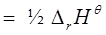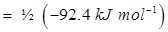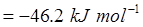### Thermodynamics - NCERT Solutions

CBSE Class 11 Chemistry

NCERT Solutions
Chapter 6
THERMODYNAMICS

1. Choose the correct answer. A thermodynamic state function is a quantity

(i) used to determine heat changes

(ii) whose value is independent of path

(iii) used to determine pressure volume work

(iv) whose value depends on temperature only.

Ans. A thermodynamic state function is a quantity whose value is independent of a path.
Functions like pVetc. depend only on the state of a system and not on the path.
Hence, option (ii) is correct.

2. For the process to occur under adiabatic conditions, the correct condition is:

(i) ΔT = 0

(ii) Δp = 0

(iii) q = 0

(iv) w= 0

Ans. A system is said to be under adiabatic conditions if there is no exchange of heat between the system and its surroundings. Hence, under adiabatic conditions, q = 0.
Therefore, option (iii) is correct.

3. The enthalpies of all elements in their standard states are:

(i) unity

(ii) zero

(iii) < 0

(iv) different for each element

Ans. The enthalpy of all elements in their standard state is zero.
Therefore, option (ii) is correct.

4. ΔU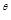¸of combustion of methane is - XkJ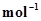. The value of ΔH¸is

(i) = ΔU(ii) > ΔU(iii) < ΔU(iv) = 0

Ans. Since ΔH= ΔU+ Δ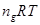and ΔU= -XkJ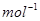,
ΔH= (-X) +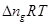.
⇒ ΔH< ΔUTherefore, option (iii) is correct.

5. The enthalpy of combustion of methane, graphite and dihydrogen at 298 K are, -890.3 kJ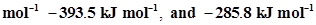respectively. Enthalpy of formation of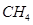(g) will be

(i) -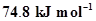(ii)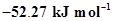(iii)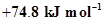(iv)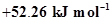.

Ans. According to the question,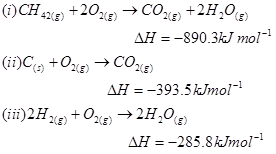Thus, the desired equation is the one that represents the formation of CH4 (g)i.e.,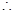Enthalpy of formation of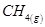=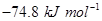Hence, option (i) is correct.

6. A reaction, A + B → C + D + is found to have a positive entropy change. The reaction will be

(i) possible at high temperature

(ii) possible only at low temperature

(iii) not possible at any temperature

(iv) possible at any temperature

Ans. For a reaction to be spontaneous, Δshould be negative.

ΔG= ΔHTΔS

According to the question, for the given reaction,

ΔS= positive

ΔH= negative (since heat is evolved)

⇒ ΔG= negative

Therefore, the reaction is spontaneous at any temperature.

Hence, option (iv) is correct.

7. In a process, 701 J of heat is absorbed by a system and 394 J ofwork is done by the system. What is the change in internal energy for the process?

Ans. According to the first law of thermodynamics,

ΔUqW (i)

Where,

ΔU= change in internal energy for a process

q= heat

W= work

Given,

q= + 701 J (Since heat is absorbed)

W= -394 J (Since work is done by the system)

Substituting the values in expression (i), we get

ΔU= 701 J + (-394 J)

ΔU= 307 J

Hence, the change in internal energy for the given process is 307 J.

8. The reaction of cyanamide,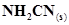,with dioxygen was carried out in a bomb calorimeter, and ΔU was found to be -742.7 kJat 298 K. Calculate enthalpy change for the reaction at 298 K.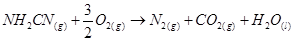Ans. Enthalpy change for a reaction (ΔH) is given by the expression,

ΔH = ΔU +Where,

ΔU = change in internal energy

Δng = change in number of moles

For the given reaction,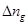=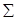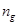(products) -(reactants)

= (2 - 1.5) moles= 0.5 moles

And,

ΔU = -742.7 kJT = 298 K

R =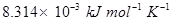Substituting the values in the expression of ΔH:

ΔH =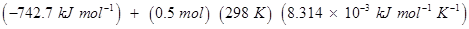= -742.7 + 1.2

ΔH = -741.5 kJ mol-1

9. Calculate the number of kJ of heat necessary to raise the temperatureof 60.0 g of aluminium from 35°C to 55°C. Molar heat capacity of Al is 24 J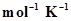.

Ans. From the expression of heat (q),

q = m. c. ΔT

Where,

c = molar heat capacity

m = mass of substance

ΔT = change in temperature

Substituting the values in the expression of q: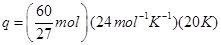q = 1066.7 J

q = 1.0667 KJ = 1.067 KJ

10. Calculate the enthalpy change on freezing of 1.0 mol of water at 10.0°C to ice at -10.0°C.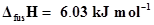at 0°C.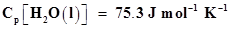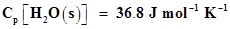Ans. According to the question,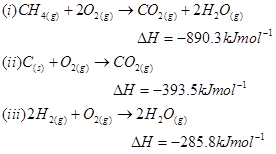Thus, the desired equation is the one that represents the formation of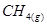i.e,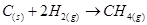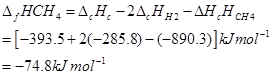Total enthalpy change involved in the transformation is the sum of the following changes:

(a) Energy change involved in the transformation of 1 mol of water at 10°C to 1 mol of water at 0°C.

(b) Energy change involved in the transformation of 1 mol of water at 0° to 1 mol of ice at 0°C.

(c) Energy change involved in the transformation of 1 mol of ice at 0°C to 1 mol of ice at-10°C.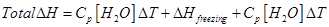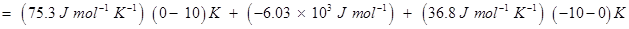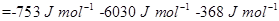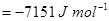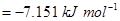Hence, the enthalpy change involved in the transformation is -7.151 kJ mol-1

11. Enthalpy of combustion of carbon to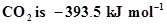. Calculate the heat released upon formation of 35.2 g of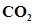from carbon and dioxygen gas.

Ans. Formation of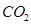from carbon and dioxygen gas can be represented as: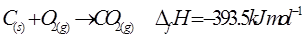(1 mole = 44 g)

Heat released on formation of 44 g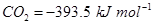Heat released on formation of 35.2 g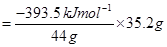=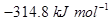12. Enthalpies of formation of CO(g),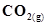,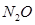(g) and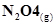are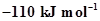,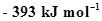,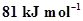and 9.7 kJrespectively. Find the value of Δ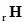for the reaction: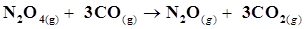Ans. Δ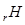for a reaction is defined as the difference between ΔfH value of products and ΔfH value of reactants.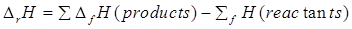For the given reaction,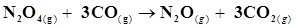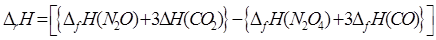Substituting the values of Δ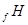for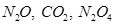,and CO from the question, we get: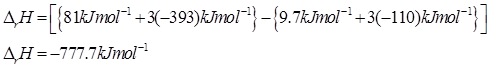Hence, the value of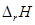for the reaction is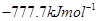.

13. Given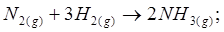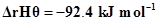What is the standard enthalpy of formation of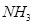gas?

Ans. Standard enthalpy of formation of a compound is the change in enthalpy that takes place during the formation of 1 mole of a substance in its standard form from its constituent elements in their standard state.

Re-writing the given equation for 1 mole of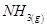,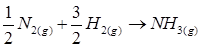Standard enthalpy of formation of